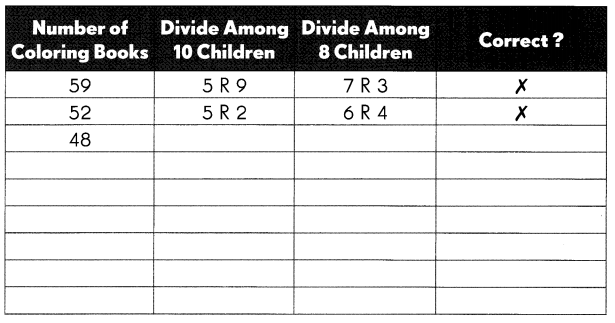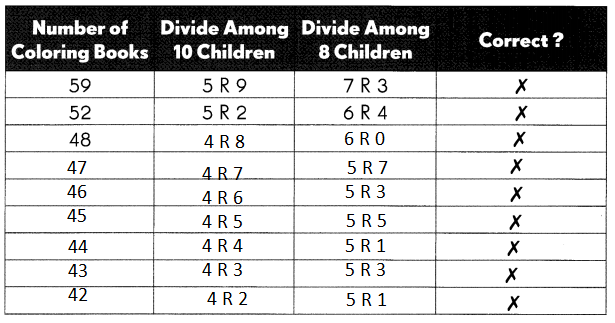Practice the problems of Math in Focus Grade 3 Workbook Answer Key Chapter 8 Division to score better marks in the exam.

Math Journal

Write True or False.

If the statement is false, rewrite the sentence to make it true.

Question 1.
When I divide one number by another, the answer is called a remainder. Any number left is called a quotient.
Explanation:
the answer is called a quotient. Any number left is called a remainder.

Question 2.
When an odd number is divided by 2, there is no remainder.
Explanation:
When an odd number is divided by 2, there is will be a remainder.

Question 3.
When an even number is divided by 2, there is a remainder.
Explanation:
The statement is true

Question 4.
I always divide the ones first, then the tens for the following: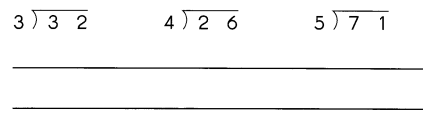no
Explanation: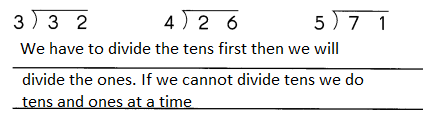Challenging Practice

Solve.

Question 1.
Find the sum of all the odd numbers between 60 and 66.
Between 60 to 66
the odd numbers are 61,63,65
Explanation:
61 +63 + 65 = 189
the sum of all the odd numbers between 60 and 66 = 189

Question 2.
Which of these division statements are true? Color them.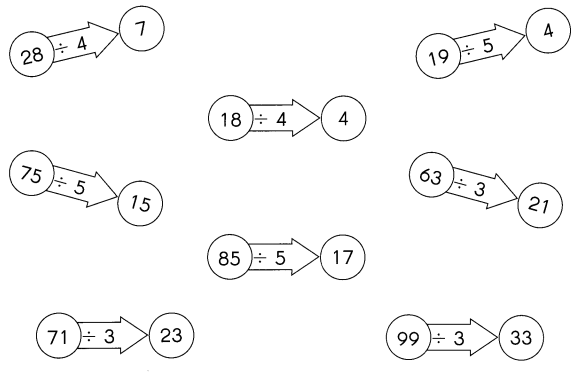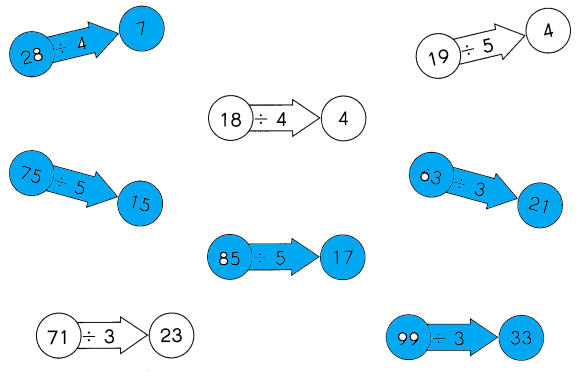Explanation:
Colored the division statement which is true

Problem Solving

Solve.

Mrs. Jones has some coloring books to give away as gifts. She has fewer than 60 but more than 40 coloring books. She will have 2 coloring books left over if she divides them equally among 10 children. She will also have 2 coloring books left over if she divides them equally among 8 children. How many coloring books does she have?